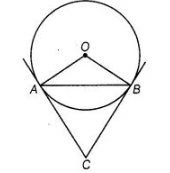# If a chord AB subtends an angle of 60°

Question:

If a chord AB subtends an angle of 60° at the centre of a circle, then angle between the tangents at A and B is also 60°.

Solution:

False

Since a chord AB subtends an angle of 60° at the centre of a circle.i.e. $\quad \angle A O B=60^{\circ}$

As $O A=O B=$ Radius of the circle

$\therefore \quad \angle O A B=\angle O B A=60^{\circ}$

The tangent at points $A$ and $B$ is drawn, which intersect at $C$. We know, $O A \perp A C$ and $O B \perp B C$.

$\therefore \quad \angle O A C=90^{\circ}, \angle O B C=90^{\circ}$

$\Rightarrow \quad \angle O A B+\angle B A C=90^{\circ}$

and $\quad \angle O B A+\angle A B C=90^{\circ}$

$\Rightarrow \quad \angle B A C=90^{\circ}-60^{\circ}=30^{\circ}$

and $\quad \angle A B C=90^{\circ}-60^{\circ}=30^{\circ}$

$\ln \Delta A B C, \quad \angle B A C+\angle C B A+\angle A C B=180^{\circ}$

[since, sum of all interior angles of a triangle is $180^{\circ}$ ]

$\Rightarrow \quad \angle A C B=180^{\circ}-\left(30^{\circ}+30^{\circ}\right)=120^{\circ}$